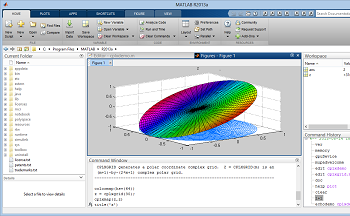# What is MATLAB?

Instructor: Sudha Aravindan

Sudha Aravindan has taught high school Math and professional development in Information Technology for over 10 years. Sudha has a Doctorate of Education degree in Mathematics Education from the University of Delaware, USA, a Masters degree in English Literature from the University of Kerala, India, a Bachelor of Education degree in Teaching of Math from the University of Kerala, India, and a Bachelor of Science degree in Math, Physics and Statistics from the University of Kerala, India. Sudha has a certificate in Java programming and Statistical Analysis.

This lesson will define MATLAB and talk about this software program's uses and usability. We'll discuss the MATLAB environment, programming language, graphing and math function, and API.

## Definition

You must have seen graphs tracking hurricanes and pie charts depicting population samples across the globe. When there is a large amount of scientific data to analyze, one of the methods to organize it and create a visual representation is by using software called matrix laboratory, or MATLAB.

MATLAB is a computer programming language that uses computations and algorithms to analyze large amounts of data and present it in visually appealing formats. Some features of MATLAB include:

• computation of numeric data
• creating graphics for scientific use
• modeling and simulating data
• analyzing data

MATLAB is a software system that has many components. Let's go over some of them here.

## MATLAB Environment

The MATLAB environment is the way the program presents itself and the tools available. The MATLAB environment allows you to run commands, manage your files, and even analyze data. It has a command window where you can write simple commands. The command window can be thought of as similar to a high performing calculator. For example, you can type in a formula (5+2)*3 and click the Execute button and MATLAB will display the result.

The MATLAB work environment provides tools that allow you to run and debug programs, install add-ons, change fonts and displays, and has functions that you can call to perform common tasks. For example, if you have the coordinate points x and y, you can use the MATLAB plot function to create a graph using the values of x and y.## MATLAB Programming Language

The MATLAB programming language is simpler than most programming languages and easier to learn. It is known as a high-level language because it is closer to the human language than the computer or machine language.

• The semi-colon in MATLAB indicates the end of statement. It can also be used to stop a statement from executing. For example, if you type in x=5+3 without the semicolon and click the Execute button, MATLAB will display the result as x=8. If you type in x=5+3; with the semicolon, and click Execute, MATLAB will not display the result of the computation.
• The % sign is used to indicate that the text following is a comment and not to be interpreted by MATLAB. Programmers use comments to provide explanations about the code they are writing. For example, in MATLAB you can write a=b+5 and the use the % sign to explain that 'a' is the length of a room and 'b' is the width.
• Variable names in MATLAB are case sensitive. For example, if you create a variable 'TempEveryHour' to represent the temperatures every hour, and need it to use this variable in a mathematical formula, you would need to call the variable by its exact same name: TempEveryHour and not TemperatureEveryHour or tempeveryhour.

## Graphs and Math Functions

MATLAB has inbuilt functions to create high quality 3-D graphs that helps us to visualize data. The mesh function creates a graph that looks like a 3-D wire mesh. And the contour function plots a graph with contour lines. The slices and points on the graph can be color coded to make the graph easier to understand and model the data in real time.

MATLAB has a large collection of mathematical functions that can be used to perform complex mathematical and scientific computations. For example, the sqrt function calculates the square root and the avg function can be used to calculate the average.

To unlock this lesson you must be a Study.com Member.

### Register to view this lesson

Are you a student or a teacher?